Home > INT3 > Chapter 7 > Lesson 7.2.1 > Problem7-57

7-57.
1.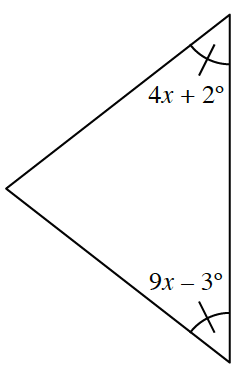Multiple Choice: Based on the relationships provided in the diagram, which of the equations below is correct? Justify your choice. Homework Help ✎

1. 4x + 2º + 9x – 3º = 90º

2. 4x + 2º = 9x – 3º

3. 4x + 2º + 9x – 3º = 180º

4. (4x + 2º)(9x – 3º) = 90º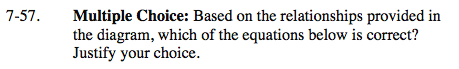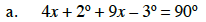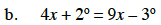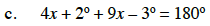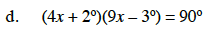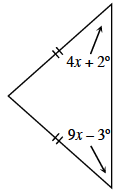This is an isosceles triangle because it has two congruent sides.
In an isosceles triangles base angles are equal.